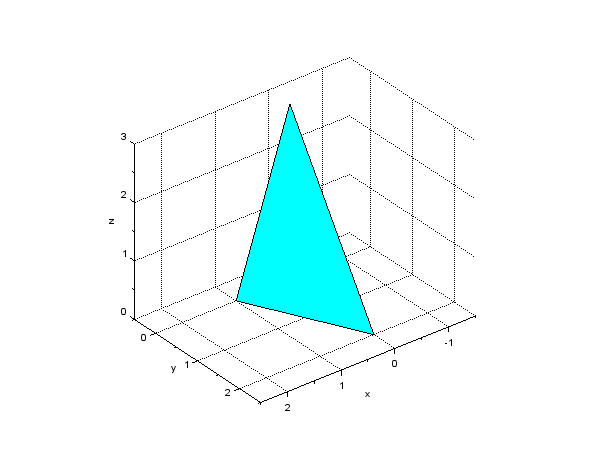Home > Resources > Making Animation with Scilab > 4. Creating polygons with plot3d
Making Animation with Scilab

## 4. Creating polygons with plot3d

To make an animation of a rigid body, we first have to create a shape that represents the rigid body. In Scilab we can use `plot3d` command to create an arbitrary three-dimensional shape. The `plot3d` command creates one or more polygonal surfaces (patches) defined by three or more vertices. By combining patches we can create an arbitrary polyhedron.

For example, Code 1 is a Scilab script that draws a triangle patch with three vertices at (1,0,0), (0,2,0), and (0,0,3). The result of the drawing by this script is shown in Figure 1.

```// test_patch.sce

clear; xdel(winsid());

// Define vertices
vertices = [
1, 0, 0;  // vertice #1
0, 2, 0;  // vertice #2
0, 0, 3]; // vertice #3

// Draw patch
h_fig = figure;
h_fig.background = 8;
plot3d(vertices(:,1), vertices(:,2), vertices(:,3));

// Axes settings
xlabel("x"); ylabel("y"); zlabel("z");
h_axes = gca();
h_axes.isoview = "on";
h_axes.box = "off";
h_axes.rotation_angles = [59, 52];
h_axes.data_bounds = [-1.5, -0.5, 0; 2.5, 2.5, 3];
xgrid;
```
Code 1: Example Scilab script that draws a triangle patch with vertices at (1,0,0), (0,2,0), (0,0,3)Figure 1: A triangle patch with vertices at (1,0,0), (0,2,0), (0,0,3)